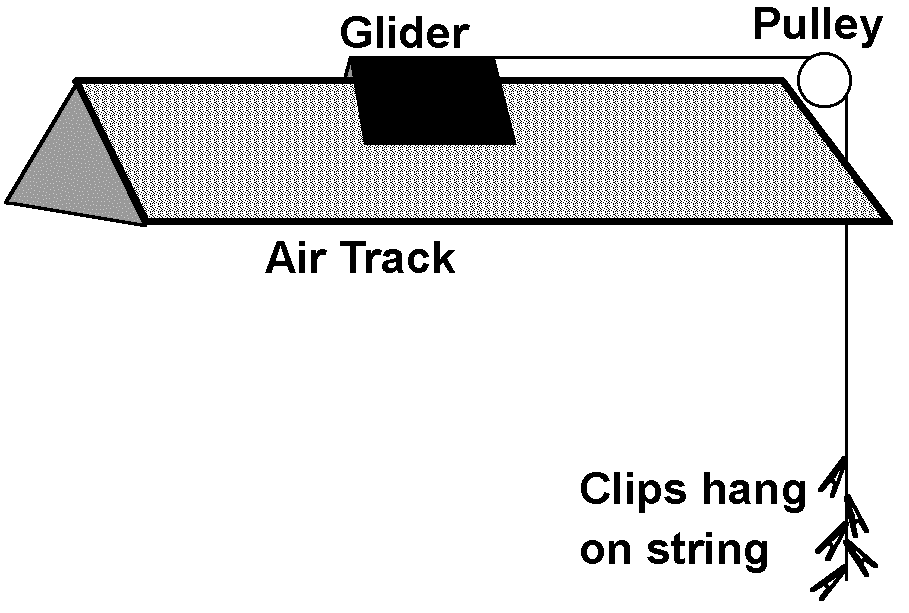# Experiment of The Month

## Newton's First and Second Laws

Dr. Miziumski uses this experiment to investigate Newton's first and second laws in our Physics 103 course for non-science majors.The experiment uses an air track (one at each of seven lab benches) to avoid a discussion of friction. The final set up, to study the second law, is sketched at the right.

The initial set-up looks the same, except that the glider is attached to nothing. With this situation, we regard the first law as a promise that you can find an inertial frame of reference. In such a frame of reference, an object with no force on it will move at constant velocity. (The velocity might be zero, of course, and the results would still be consistent with the first law.)

The air track is leveled with its leveling screws. The test of "level" is to push the glider gently and measure its velocity as it travels the length of the air track. If the velocity is constant, then we can say two things:

1. We are in an inertial frame of reference.
2. There is no horizontal force on the glider.

With this level situation established, we add exactly one force to the glider, using the string, hanging over the pulley, weighted by small alligator clips. (The clips are called "micro alligator clips," and have a mass of about 1 gram per clip.) The clips provide a downward pull on the string which translates into a horizontal force on the glider. When the clips are attached, the glider is accelerated to the right.

If there were no acceleration, each clip would pull down on the string with a force equal to its weight. In the experiment, of course, there is acceleration, but it is on the order of 1% of the gravitational field strength (the acceleration due to gravity of a free falling object) and we can safely ignore this complication.

We argue that 2 clips pull down twice as hard as 1, that 3 pull down three times as hard as 1 clip, etc. The test of Newton's second law is then done as follows:

1. Measure the position as a function of time for 3 clips. ( In our experience, 1 clip cannot reliably overcome the small friction that the glider experiences. Dr. Miziumski has written a handy computer program for PC's that records the time each time the space bar on the keyboard is pressed. We use this to measure the time when the glider passes marks that we have put on the air track.)
2. Calculate the average velocity between pairs of measured positions, using the measured time interval.
3. Plot the velocity as a function of time and use a straight line through the points to estimate the slope (which is the acceleration of the glider).
4. Plot the acceleration versus number of clips. Newton's second law predicts that the plot will be a straight line.

In this course, we do not venture into detailed calculation of the acceleration in terms of the mass of a clip and the mass of the glider. The point is that we can understand the mathematical relation by studying the shape of the graph. We do not need numbers for the force in order to test the idea of Newton's second law.

An interesting variation is to give the glider a (small) initial velocity and observe that the acceleration is the same as when the initial velocity was zero. This observation justifies being less than particular about the initial velocity of the glider, when the clips are allowed to begin pulling it down.

## Experiment Of The Month

View past labs experiments.